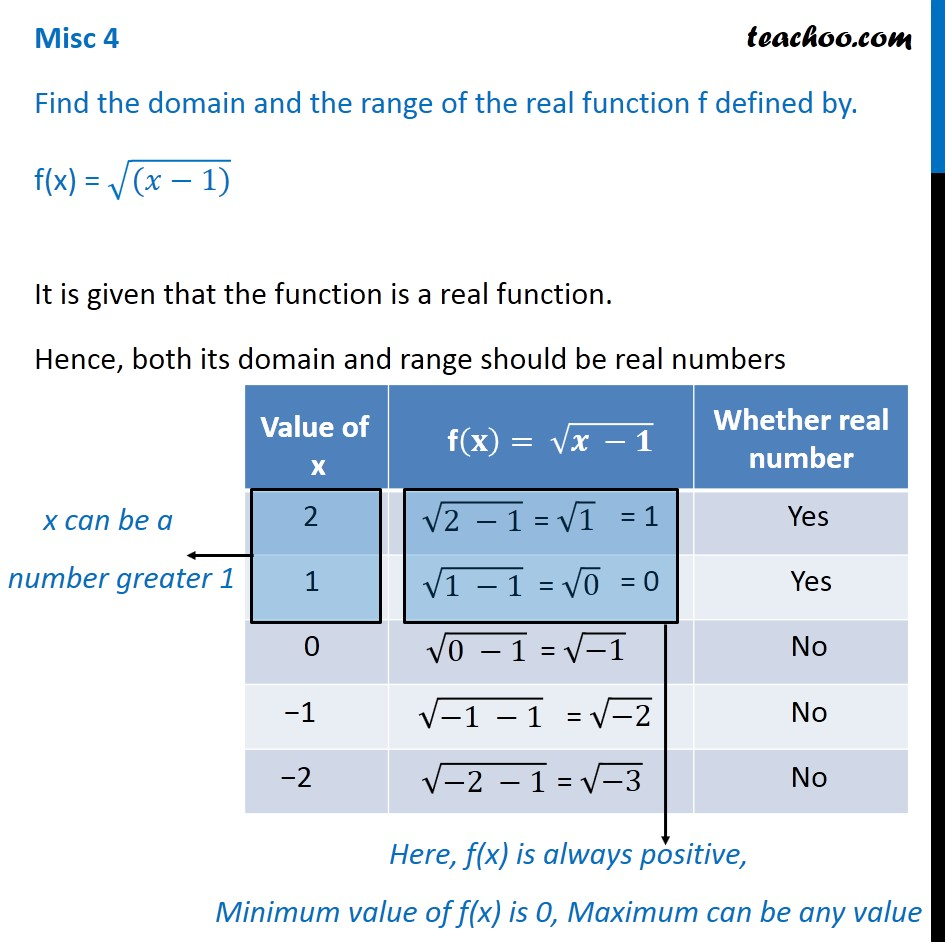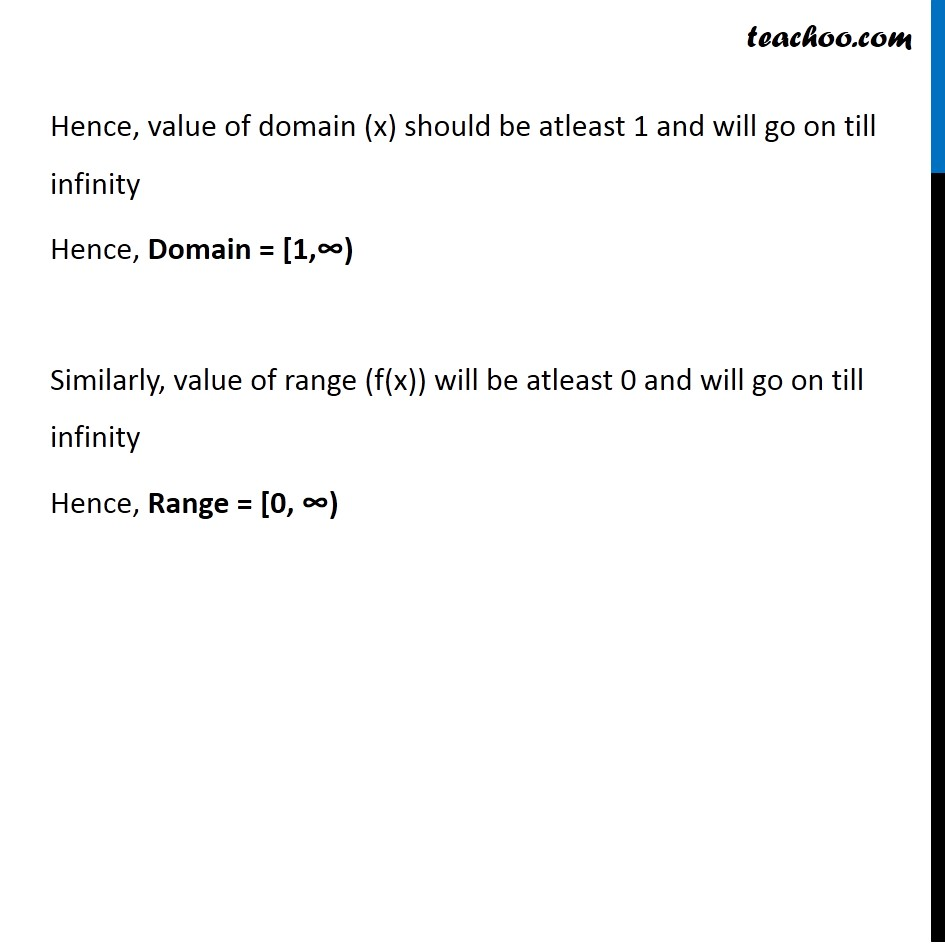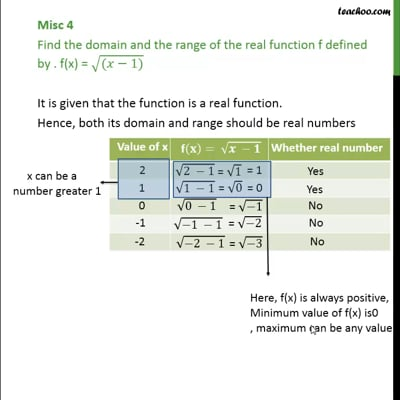Miscellaneous

Chapter 2 Class 11 Relations and Functions
Serial order wiseThis video is only available for Teachoo black users

Learn in your speed, with individual attention - Teachoo Maths 1-on-1 Class

### Transcript

Misc 4 Find the domain and the range of the real function f defined by. f(x) = √((𝑥−1)) It is given that the function is a real function. Hence, both its domain and range should be real numbers x can be a number greater 1 Here, f(x) is always positive, Minimum value of f(x) is 0, Maximum can be any value Hence, value of domain (x) should be atleast 1 and will go on till infinity Hence, Domain = [1,∞) Similarly, value of range (f(x)) will be atleast 0 and will go on till infinity Hence, Range = [0, ∞)